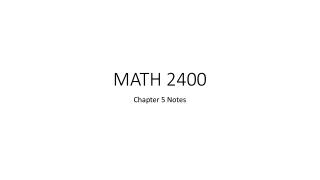DownloadDownload PresentationMATH 2400

# MATH 2400

Télécharger la présentation## MATH 2400

- - - - - - - - - - - - - - - - - - - - - - - - - - - E N D - - - - - - - - - - - - - - - - - - - - - - - - - - -
##### Presentation Transcript

1. MATH 2400 Chapter 5 Notes

2. Regression Line Uses data to create a linear equation in the form y = ax + b where “a” is the slope of the line (unit rate of change) “b” is the y-intercept (initial value) Can be used generalize a set of data, to estimate a value, or predict a value.

3. Example 1 (Exercise 5.1) We expect a car’s highway gas mileage to be related to its city gas mileage. Data for all 1040 vehicles in the government’s 2010 Fuel Economy Guide give the regression line HWY MPG = 6.554 + (1.016 x CITY MPG) for predicting highway mileage from city mileage. • What is the slope of this line? Say in words what the numerical value of the slope tells you. • What is the intercept? Explain why the value of the intercept is not statistically meaningful. • Find the predicted highway mileage for a car that gets 16 mpg in the city. Do the same for a car with city mileage 28 mpg.

4. Example 2 (Exercise 5.2…sort of) You use the same bottle of body wash every day. The volume was initially 355 ml. What is the equation of the regression line for predicting the volume of body wash left in the bottle after each day?

5. Least-Square Regression Line Where and . Sy represents the standard deviation of the response variable. Sx represents the standard deviation of the explanatory variable. r represents the correlation coefficient. represents the mean of the explanatory variable. represents the mean of the response variable.

6. Example 3 This table displays the data regarding 8 U.S airports and their total number of passengers for the year 1992 and 2005. Use the 1992 data as the explanatory variable and the 2005 data as the response variable. Create a least-squares regression line and use that line to estimate how many passengers Raleigh-Durham International had in 2005 if the airport had 4.9 million passengers in1992.

7. r and r2 • r tells us if there is a positive or negative relationship between the explanatory variable and theresponse variable. • r also tells us how strong of a relationship the variables have. • r2 tells us what portion of the linear relationship between the variables can be explained by the explanatory variable. • 1 – r2 tells us what portion of the linear relationship between the variables can not be explained by the explanatory variable. Ex: If r = 0.6,  r2 = 0.36. 36% of the linear relationship can be explained by the explanatory variable and 64% cannot be explained. Ex: If r = -1,  r2 = 1. 100% of the linear relationship can be explained by the explanatory variableand 0% cannot be explained.

8. Example 4

9. Example 5

10. Residuals A residual is the difference between an observed value of the response variable and the value predicted by the regression line. That is, a residual is the prediction error that remains after we have chosen the regression line: Residual = observed y – predicted y = y -

11. Residuals…continued A residual plot makes it easier to see unusual observations and patterns. The regression line is horizontal (think about it…).

12. Residual Graphing Use the following data to create a least-squares regression line and plot the residuals on the graph provided.

13. CAUTION!!! • Correlation and regression lines describe only linear relationships. • Correlation and least-squares regression lines are not resistant to influential data (data drastically outside the norm). We should always plot our data and look for observations that might be influential. • Ecological Correlation is based on averages rather than on individuals. Ex: There is a large positive correlation between average income and number of years of education. The correlation is smaller if we compare the incomes of individuals with number of years of education. The correlation based on average income ignores the large variation in the incomes of individuals having the same amount of education.

14. CAUTION!!! Extrapolation is the use of a regression line for prediction far outside the range of values of the explanatory variable that you used to obtain the line. Ex: Using the least-squares regression line for the height of the child from ages 0-9 to predict their height at age 30. Lurking Variables should always be thought about before drawing conclusions based on correlation or regression.

15. Correlation  Causation??? NO!!! A serious study once found that people with two cars live longer than people who own only one car. Owning three cars is even better, and so on. There is a substantial positive correlation between number of cars x and length of life y. Lurking variables?

16. HW 5.17

17. HW 5.25

18. HW 5.27

19. HW 5.29

20. HW 5.53